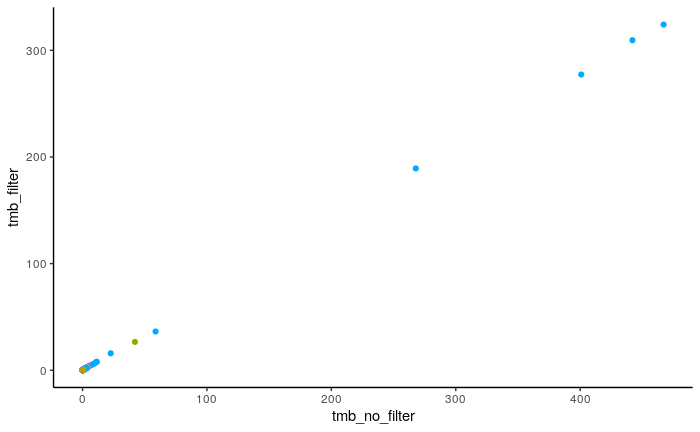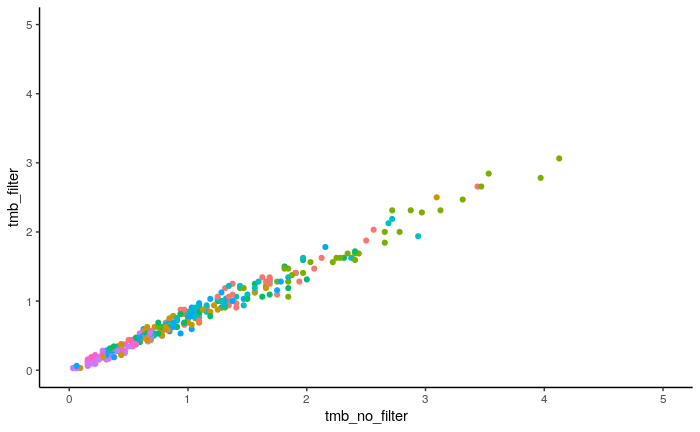### Summary of Findings:

This was an exploration of how TMB is affected by using nonsynonymous filters. It addresses part 1 of this OpenPBTA issue As far as the TMB comparisons go, it doesn’t matter if we use `Variant_Classification` filters or not. They are highly correlated no matter what and the same general TCGA to PBTA differences seem to persist.

### Usage

If both `run_caller_consensus_analysis-tcga.sh` and `run_caller_consensus_analysis-pbta.sh` have been run, you can run this command. This will prepare the files needed for this notebook and run this notebook:

``# bash run_explorations.sh``

## Setup

``````# Magrittr pipe
`%>%` <- dplyr::`%>%```````
``source(file.path("..", "..", "tmb-compare", "util", "cdf-plot-function.R"))``
``dir.create("plots", showWarnings = FALSE)``

## Read in the TMB files

Import the tmb calculations that are not filtered by nonsynonymous classifications.

``````tmb_pbta_no_filter <- data.table::fread(file.path(
"..",
"results",
"no_filter",
"pbta-snv-mutation-tmb-coding.tsv"
)) %>%
dplyr::filter(experimental_strategy != "Panel") %>%
dplyr::mutate(filter = "no filter")``````

Repeat same steps for original tmb calculations (with filter).

``````tmb_pbta_with_filter <- data.table::fread(file.path(
"..",
"results",
"consensus",
"pbta-snv-mutation-tmb-coding.tsv"
)) %>%
dplyr::filter(experimental_strategy != "Panel") %>%
dplyr::mutate(filter = "filter")``````

Combine the unfilter and filtered data sets into one.

``````tmb_pbta <- dplyr::inner_join(tmb_pbta_no_filter,
dplyr::select(tmb_pbta_with_filter, Tumor_Sample_Barcode, tmb),
by = "Tumor_Sample_Barcode",
suffix = c("_no_filter", "_filter")
)``````

Repeat the same steps for the TCGA data.

``````tmb_tcga_no_filter <- data.table::fread(file.path(
"..",
"results",
"no_filter",
"tcga-snv-mutation-tmb-coding.tsv"
)) %>%
dplyr::mutate(filter = "no filter")``````
``````tmb_tcga_with_filter <- data.table::fread(file.path(
"..",
"results",
"consensus",
"tcga-snv-mutation-tmb-coding.tsv"
)) %>%
dplyr::mutate(filter = "filter")``````

Join these together.

``````tmb_tcga <- dplyr::inner_join(tmb_tcga_no_filter,
dplyr::select(tmb_tcga_with_filter, Tumor_Sample_Barcode, tmb),
by = "Tumor_Sample_Barcode",
suffix = c("_no_filter", "_filter")
)``````

## Does the filter change a participant’s TMB?

### Plot PBTA data

Correlate first with Pearson’s.

``cor.test(tmb_pbta\$tmb_filter, tmb_pbta\$tmb_no_filter)``
``````
Pearson's product-moment correlation

data:  tmb_pbta\$tmb_filter and tmb_pbta\$tmb_no_filter
t = 2488.9, df = 934, p-value < 2.2e-16
alternative hypothesis: true correlation is not equal to 0
95 percent confidence interval:
0.9999143 0.9999337
sample estimates:
cor
0.9999246 ``````
``````pbta_cor_plot <- ggplot2::ggplot(
tmb_pbta,
ggplot2::aes(x = tmb_no_filter, y = tmb_filter, color = short_histology)
) +
ggplot2::geom_point() +
ggplot2::theme_classic() +
ggplot2::theme(legend.position = "none")

pbta_cor_plot``````This data is very skewed, let’s try a nonparametric correlation.

``cor.test(tmb_pbta\$tmb_filter, tmb_pbta\$tmb_no_filter, method = "spearman")``
``Cannot compute exact p-value with ties``
``````
Spearman's rank correlation rho

data:  tmb_pbta\$tmb_filter and tmb_pbta\$tmb_no_filter
S = 3031423, p-value < 2.2e-16
alternative hypothesis: true rho is not equal to 0
sample estimates:
rho
0.9778195 ``````

Still pretty good.

### Plot TCGA data

``cor.test(tmb_tcga\$tmb_filter, tmb_tcga\$tmb_no_filter)``
``````
Pearson's product-moment correlation

data:  tmb_tcga\$tmb_filter and tmb_tcga\$tmb_no_filter
t = 4296.2, df = 316, p-value < 2.2e-16
alternative hypothesis: true correlation is not equal to 0
95 percent confidence interval:
0.9999893 0.9999931
sample estimates:
cor
0.9999914 ``````
``````tcga_cor_plot <- ggplot2::ggplot(
tmb_tcga,
ggplot2::aes(x = tmb_no_filter, y = tmb_filter, color = short_histology)
) +
ggplot2::geom_point() +
ggplot2::xlim(0, 5) +
ggplot2::ylim(0, 5) +
ggplot2::theme_classic() +
ggplot2::theme(legend.position = "none")

tcga_cor_plot``````Correlate with nonparametric correlation since we did that for PBTA.

``cor.test(tmb_tcga\$tmb_filter, tmb_tcga\$tmb_no_filter, method = "spearman")``
``Cannot compute exact p-value with ties``
``````
Spearman's rank correlation rho

data:  tmb_tcga\$tmb_filter and tmb_tcga\$tmb_no_filter
S = 60671, p-value < 2.2e-16
alternative hypothesis: true rho is not equal to 0
sample estimates:
rho
0.9886798 ``````

## Are some histologies affected more than others?

Let’s make the same plots but by `short_histology`.

Plotting PBTA data, but setting the scales to “free” since the histologies have very different tmb ranges.

``````pbta_cor_plot +
ggplot2::facet_wrap(~short_histology, scales = "free")``````